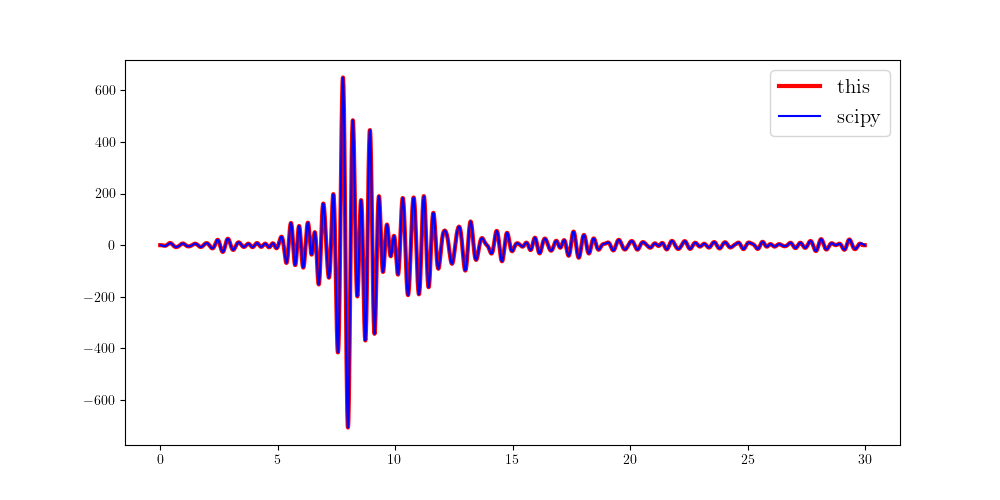## 1 Basic princeples

For a Butterworth high-pass filter, the squared frequency response is given by $$|H(\omega)|^2 = \frac{1}{1+(\frac{tan\frac{\omega_c}{2}}{\frac{\omega}{2}})^{2N}} \tag{1}.$$ Using $tan\frac{\omega}{2}=\frac{1}{j}\frac{1-e^{-j\omega}}{1+e^{-j\omega}}$ and $z=e^{-j\omega}$, we have $$|H(z)|^2=\frac{(-1)^N(\frac{1-z^{-1}}{1+z^{-1}})^{2N}}{(-1)^N(\frac{1-z^{-1}}{1+z^{-1}})^{2N}+tan^{2N}\frac{\omega_c}{2}} \tag{2}.$$ We know there $N$ repeated zeros $1$ of this transfer function, and the poles are little bit complicated. We first calculate $q_k=\frac{1-z_k^{-1}}{1+z^{-1}}$. $$q_k= \begin{cases} &tan\frac{\omega_c}{2}e^{j\frac{2k+1}{2N}\pi}, \text{ for } N \text{ is even and }k=0, 1, 2, \cdots, 2N-1;\\ &tan\frac{\omega_c}{2}e^{j\frac{k}{N}\pi}, \text{ for } N \text{ is odd and }k=0, 1, 2, \cdots, 2N-1. \end{cases} \tag{3},$$ and $z_k=\frac{1+q_k}{1-q_k}$. The transfer function of a Butterworth high-pass filter is given by $$H(z)= \frac{1}{2^N}\frac{\prod_{|z_k|<1}(1+z_k)(1-z^{-1})^N}{\prod_{|z_k|<1}(1-z_kz^{-1})} \tag{4}.$$

## 2 Python code

Here, we give an example of implementing the Butterworth high-pass filter and compare it with that generated by scipy.

  1 2 3 4 5 6 7 8 9 10 11 12 13 14 15 16 17 18 19 20 21 22 23 24 25 26 27 28 29 30 31 32 33 34 35 36 37 38 39 40 41 42 43 44 45 46 47 48 49 50 51 52  import numpy as np import matplotlib.pyplot as plt from obspy import read from scipy.signal import butter, filtfilt from scipy import fft def highpass(d, fs, fc, orders=4, zerophase=False, taper=0.01): n = len(d) f = np.arange(n) * fs / n w = 2 * np.pi * f / fs wc = 2 * np.pi * fc / fs k = np.arange(2*orders) if orders % 2 == 0: q = np.tan(wc/2) * np.exp(1j*(k+0.5)/orders*np.pi) else: q = np.tan(wc/2) * np.exp(1j*k/orders*np.pi) p = (1 + q) / (1 - q) z = np.exp(1j*w) h = np.ones_like(w, dtype=complex) for pp in p[abs(p)<1]: h *= ((1+pp) / (1-pp/z)) h *= ((1-1/z) ** orders / 2**orders) dd = fft.ifft(fft.fft(d) * h).real if zerophase: dd = fft.ifft(fft.fft(dd[::-1])*h)[::-1].real ni = int(n*taper) k1 = np.arange(ni) k2 = (-k1)[::-1] ta1 = np.cos(np.pi*k1/ni/2) ** N ta2 = np.cos(np.pi*k2/ni/2) ** N dd[:ni] *= ta2 dd[-ni:] *= ta1 return f, h, dd tr = read() tr.filter('bandpass', freqmin=0.5, freqmax=3, corners=4, zerophase=True) dt = tr.stats.delta fs = 1 / dt d1 = tr.data.copy() n = len(d1) t = np.arange(n) * dt fc = 1.5 N = 4 f, h, d2 = highpass(d1, fs, fc, orders=N, zerophase=True, taper=0.01) [b, a] = butter(N, 2*fc/fs, 'highpass') d3 = filtfilt(b, a, d1) plt.figure(figsize=(10, 5)) plt.plot(t, d2, 'r', lw=3, label='this') plt.plot(t, d3, 'b', lw=1.5, label='scipy') plt.legend(fontsize=15) plt.show()